## Series Connection of Coupled Inductors:

Let there be two inductors connected in series, with self inductances L1 and L2 and mutual inductance of M. Two kinds of Series Connection of Coupled Inductors are possible; series aiding as in Fig. 10.19(a), and series opposition as in Fig. 10.19(b).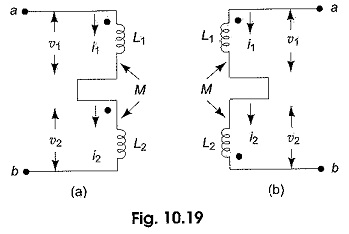In the case of series aiding connection, the currents in both inductors at any instant of time are in the same direction relative to like terminals as shown in Fig. 10.19(a). For this reason, the magnetic fluxes of self induction and of mutual induction linking with each element add together.

In the case of series opposition connection, the currents in the two inductors at any instant of time are in opposite direction relative to like terminals as shown in Fig. 10.19(b). The inductance of an element is given by L = Φ/i, where Φ is the flux produced by the inductor.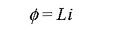For the series aiding circuit, if Φ1 and Φ2 are the flux produced by the coils 1 and 2, respectively, then the total flux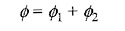where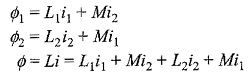Since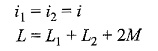Similarly, for the series opposition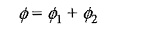where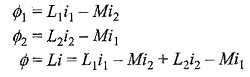Since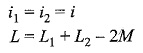In general, the inductance of Series Connection of Coupled Inductors is given by L = L1 + L2 ± 2M.
Positive sign is applied to the series aiding connection, and negative sign to the series opposition connection.

Scroll to Top